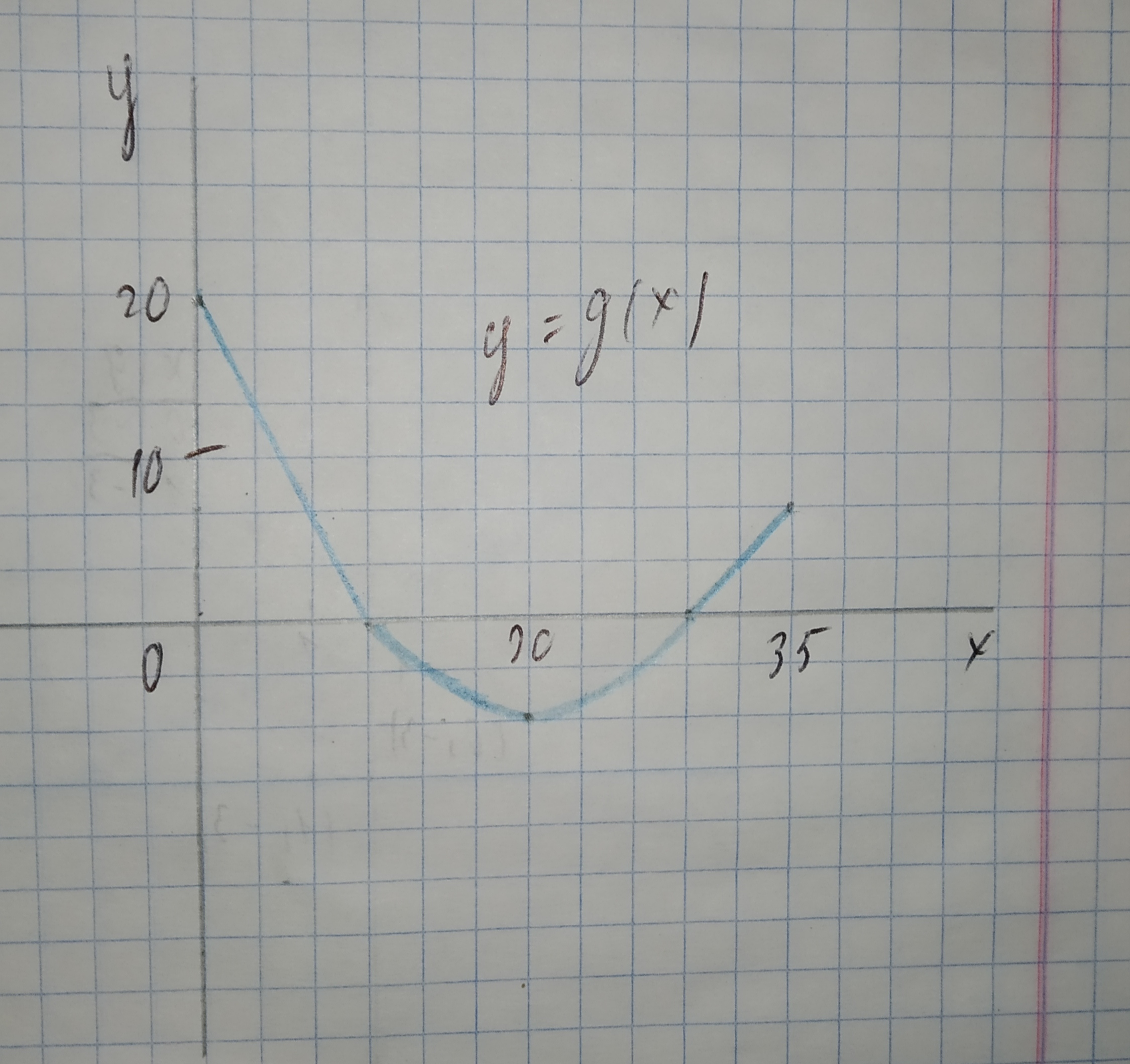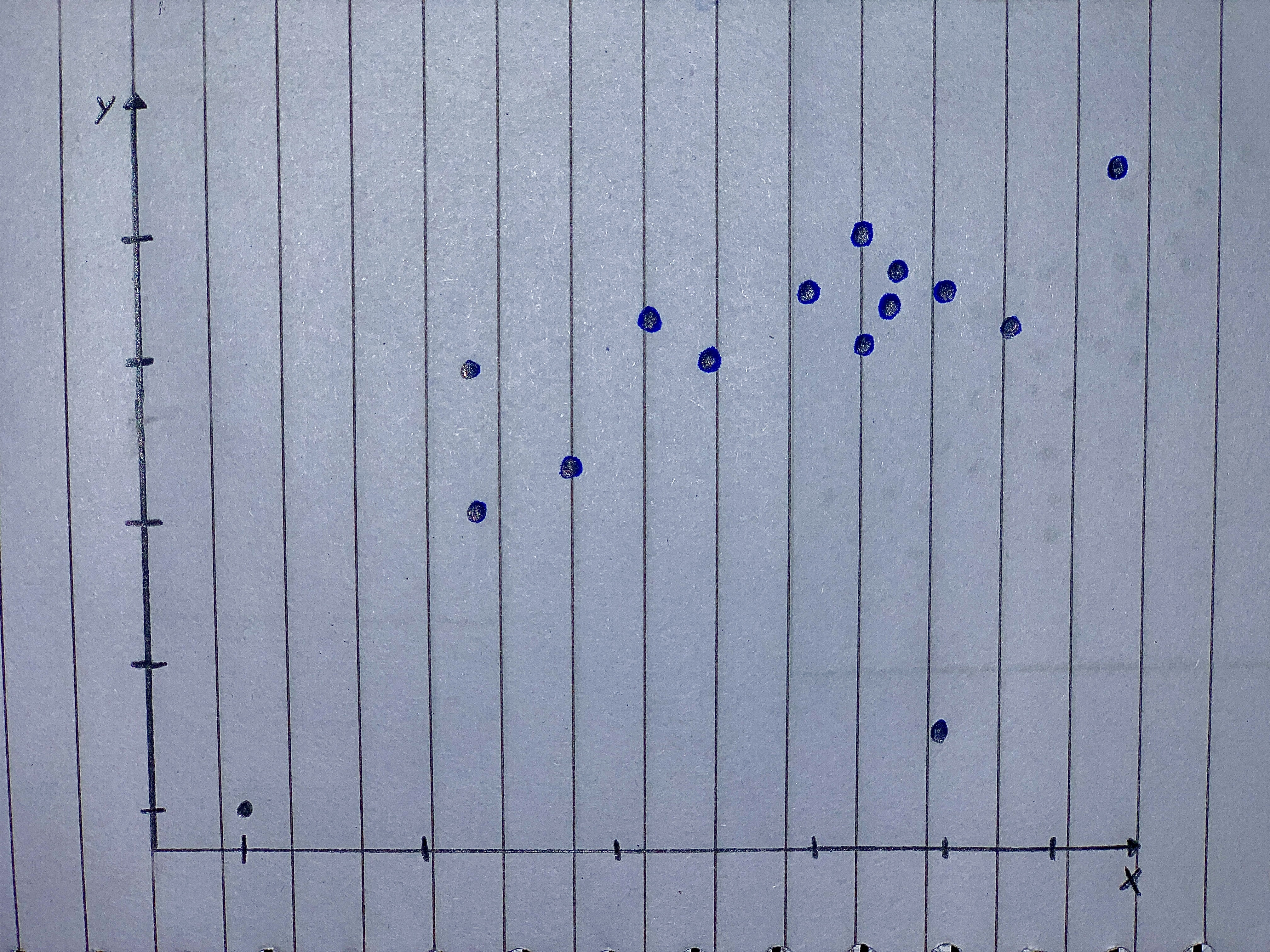# The graph of g consists of two straight lines and a semicircle. Use it to eveluate the integral. 12210203861.jpg int_0^35 g(x)dxQuestion
Applications of integralsThe graph of g consists of two straight lines and a semicircle. Use it to eveluate the integral.$$\displaystyle{\int_{{0}}^{{35}}}{g{{\left({x}\right)}}}{\left.{d}{x}\right.}$$2021-02-20
The integral $$\displaystyle{\int_{{0}}^{{35}}}{g{{\left({x}\right)}}}{\left.{d}{x}\right.}$$ is the area between the graph of g and the horizontal x-axis on the interval $$\displaystyle{0}\le{x}\le{35}$$. We separate this integral into the parts of (a) and (b) along with
$$\displaystyle{\int_{{30}}^{{35}}}{g{{\left({x}\right)}}}{\left.{d}{x}\right.}$$.
$$\displaystyle{\int_{{30}}^{{35}}}{g{{\left({x}\right)}}}{\left.{d}{x}\right.}$$ represents the area that forms a triangle with base b = 5 and height h = 5.
The area of a triangle is the product of the base b and the height h, divided by 2.
$$\displaystyle{\int_{{30}}^{{35}}}{g{{\left({x}\right)}}}{\left.{d}{x}\right.}=\frac{{{b}\cdot{h}}}{{2}}$$
$$\displaystyle=\frac{{{5}\cdot{5}}}{{2}}$$
$$\displaystyle=\frac{{25}}{{2}}$$
=12.5

### Relevant QuestionsThe graph of g consists of two straight lines and a semicircle. Use it to eveluate the integral.$$\displaystyle{\int_{{0}}^{{10}}}{g{{\left({x}\right)}}}{\left.{d}{x}\right.}$$The graph of g consists of two straight lines and a semicircle. Use it to eveluate the integral.$$\displaystyle{\int_{{10}}^{{30}}}{g{{\left({x}\right)}}}{\left.{d}{x}\right.}$$Use a table of integrals to evaluate the definite integral.
$$\displaystyle{\int_{{0}}^{{3}}}\sqrt{{{x}^{{2}}+{16}}}{\left.{d}{x}\right.}$$Consider the integral as attached, To determine the convergence or divergence of the integral, how many improper integrals must be analyzed? What must be true of each of these integrals for the given integral to converge?
$$\displaystyle{\int_{{0}}^{{3}}}\frac{{10}}{{{x}^{{2}}-{2}{x}}}{\left.{d}{x}\right.}$$.Straight Lines Graph the straight lines in Exercises 1–3.
Then find the change in y for a one-unit change in x, find the point at which the line crosses the y-axis, and calculate the value of y when x 52.5.
1. y x 5 1 2.0 0.5
2. y x 5 1 40 36.2
3. y x 5 25 6
Scatterplots For the scatterplots in Exercises 5 describe the pattern that you see. How strong is the pattern? Do you see any outliers or clusters?This exercise requires the use of a graphing calculator or computer programmed to do numerical integration. The normal distribution curve, which models the distributions of data in a wide range of applications, is given by the function $$p(x)=\frac{1}{\sqrt{2 \pi}^{\sigma}}e^{-(x-\mu)^{2}}/(2 \sigma^{2})$$ where $$\pi = 3.14159265 . . .$$ and sigma and mu are constants called the standard deviation and the mean, respectively. Its graph$$(\text{for}\ \sigma=1\ \text{and}\ \mu=2)$$is shown in the figure. With $$\sigma = 5 \text{and} \mu = 0$$, approximate $$\int_0^{+\infty}\ p(x)\ dx.$$Suppose $$\displaystyle{\int_{{{5}}}^{{{6}}}}{f{{\left({x}\right)}}}{\left.{d}{x}\right.}={6}{\quad\text{and}\quad}{\int_{{{5}}}^{{{6}}}}{g{{\left({x}\right)}}}{\left.{d}{x}\right.}={2}$$.
Evaluate $$\displaystyle{\int_{{{5}}}^{{{6}}}}{\left({4}{f{{\left({x}\right)}}}-{2}{g{{\left({x}\right)}}}\right)}{\left.{d}{x}\right.}$$.
Remember to include a "+C" if appropriate.$$\displaystyle{\int_{{0}}^{{4}}}{\int_{{0}}^{{12}}}{2}{e}^{{{x}+{3}{y}}}{\left.{d}{x}\right.}{\left.{d}{y}\right.}$$Suppose f has absolute minimum value m and absolute maximum value M. Between what two values must $$\displaystyle\int{0}^{{2}}{f{{\left({x}\right)}}}{\left.{d}{x}\right.}$$ lie? Which property of integrals allows you to make your conclusion?